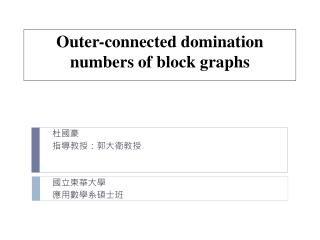DownloadDownload PresentationOuter-connected domination numbers of block graphs

# Outer-connected domination numbers of block graphs

Download Presentation## Outer-connected domination numbers of block graphs

- - - - - - - - - - - - - - - - - - - - - - - - - - - E N D - - - - - - - - - - - - - - - - - - - - - - - - - - -
##### Presentation Transcript

1. Outer-connected domination numbers of block graphs 杜國豪 指導教授：郭大衛教授 國立東華大學 應用數學系碩士班

2. Outline: • Introduction • Main result • Full k-ary tree • Block graph • Reference

3. Definition: • For a graph a set is a dominating set if . • A dominating set is an outer-connected dominating set(OCD set) if the subgraph induced by is connected. Example:

4. Definition: • For a graph a set is a dominating set if . • A dominating set is an outer-connected dominating set(OCD set) if the subgraph induced by is connected. Example:

5. Definition: • Afull -ary tree with height denoted is a k-ary tree with all leaves are at same level.

6. Proposition 1: • If is a tree and is an outer-connected dominating set of , then either or every leaf of belongs to Lemma 2: • If is a cut-vertex of and are the components of then for every outer-connected dominating set of which contains there exists such that

7. Theorem 3: For all ,

8. Theorem 4: For all

9. Definition: • A block of a graph is a maximal -connected subgraph of • A block graph is a graph which every block is a complete graph. • The block-cut-vertex tree of a graph is a bipartite graph in which one partite set consists of the cut-vertices of , and the other has a vertex for each block of And adjacent to , if containing in

10. Example:

11. Example:

12. Example:

13. Example:

14. Red: cut-vertex Blue: block Example:

15. Example:

16. Example:

17. Algorithm for block graphs:

18. Initial values: Time complexity: • Each vertex uses a constant time for computing its parameters, the time complexity of this algorithm is

19. Example 1:

20. Example 1:

21. Example 1:

22. Example 1:

23. Example 1:

24. Example 1:

25. Example 1:

26. Example 2:

27. Red: cut-vertex Blue: block Example 2:

28. Example 2:

29. Example 2:

30. Example 3:

31. Example 3: Red: cut-vertex Blue: block

32. Example 3:

33. Example 3:

34. Reference: • Akhbari, R. Hasni, O. Favaron, H. Karami and S. M. Sheikholeslami, "On the outer-connected domination in graphs," J. Combin. Optimi. DOI 10.1007/s10878-011-9427-x (2011). • J. Cyman, The outer-connected domination number of a graph, Australas. J. Combin., 38 (2007), 35-46. • H. Jiang and E. Shan, Outer-connected domination number in graph, Utilitas Math., 81 (2010), 265-274.

35. THANK YOU!